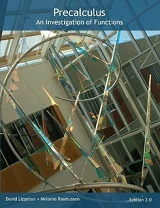Processing ......FreeComputerBooks.com Links to Free Computer, Mathematics, Technical Books all over the World

Precalculus: An Investigation of Functions

• Title Precalculus: An Investigation of Functions
• Author(s) David Lippman and Melonie Rasmussen
• Publisher: CreateSpace 2.0 Edition (2017); eBook (CC Edition 2.2, August 2020)
• License(s): CC BY-SA 3.0 US
• Paperback 374 pages (Vol 1), 344 pages (Vol 2)
• eBook PDF (704 pages), and Microsoft Word
• Language: English
• ISBN-10/ASIN: 1548407127 (Vol 1), 1548407720 (Vol 2)
• ISBN-13: 978-1548407124 (Vol 1), 978-1548407728 (Vol 2)Book Description

This book is a college level text in precalculus and trigonometry.

Problem solving and mathematical modeling are introduced early and reinforced throughout, providing students with a solid foundation in the principles of mathematical thinking. Comprehensive and evenly paced, the book provides complete coverage of the function concept, and integrates a significant amount of graphing calculator material to help students develop insight into mathematical ideas.

The first portion of the book is an investigation of functions, exploring the graphical behavior of, interpretation of, and solutions to problems involving linear, polynomial, rational, exponential, and logarithmic functions. An emphasis is placed on modeling and interpretation, as well as the important characteristics needed in calculus.

The second portion of the book introduces trigonometry. Trig is introduced through an integrated circle/triangle approach. Identities are introduced in the first chapter, and revisited throughout. Likewise, solving is introduced in the second chapter and revisted more extensively in the third chapter. As with the first part of the book, an emphasis is placed on motivating the concepts and on modeling and interpretation.

Reviews, Ratings, and Recommendations: Related Book Categories: Read and Download Links:Similar Books:
 :All CategoriesRecent BooksMiscellaneous BooksComputer LanguagesComputer ScienceData Science/DatabasesElectrical EngineeringJava and Java EE (J2EE)Linux and UnixMathematicsMicrosoft and .NETMobile ComputingNetworking and CommunicationsSoftware EngineeringSpecial TopicsWeb Programming